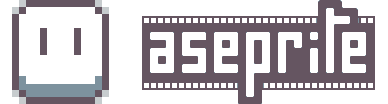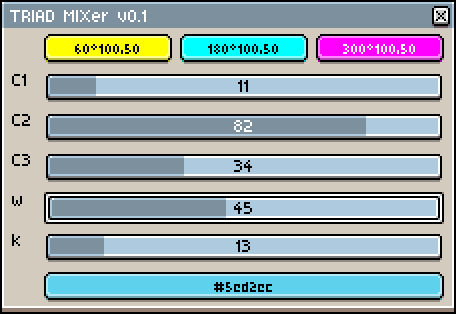# TRIAD mixer - mix colours like a painter | v0.1

mix three colours + black and white together.each slider represents an amount of ‘paint’ in the mix, so the result is ratio of all of them.
that means C1: 23 and C2: 23 will get you same mix as C1: 100 and C2: 100 etc.

note: alpha is ignored

tip: if you want to have wide range of both bright and dark mixes, make the C1 + C2 + C3 mix with lower values, so the w and k sliders can have bigger impact.
i’m thinking about replacing white and black with saturation and value for practical reasons.
we’ll see…

``````local dlgWin = Dialog{ title = "TRIAD MIXer v0.1" }

dlgWin
:color{ id = "c1",
--label = "c1",
color = Color{ h=0.0, s=1.0, l=0.5, a=255 }
}

:color{ id = "c2",
--label = "c2",
color = Color{ h=60.0, s=1.0, l=0.5, a=255 }
}

:color{ id = "c3",
--label = "c3",
color = Color{ h=210.0, s=1.0, l=0.5, a=255 }
}

:slider{ id = "c1_val",
label = "C1",
min = 0,
max = 100,
value = 0,
onchange = function()
update( dlgWin )
end
}

:slider{ id = "c2_val",
label = "C2",
min = 0,
max = 100,
value = 0,
onchange = function()
update( dlgWin )
end
}

:slider{ id = "c3_val",
label = "C3",
min = 0,
max = 100,
value = 0,
onchange = function()
update( dlgWin )
end
}

:slider{ id = "W",
label = "w",
min = 0,
max = 100,
value = 0,
onchange = function()
update( dlgWin )
end
}

:slider{ id = "K",
label = "k",
min = 0,
max = 100,
value = 0,
onchange = function()
update( dlgWin )
end
}

:color{ id = "c0",
--label = "c0",
color = Color{ h=0.0, s=0.0, l=0.5, a=255 } --app.Color
}

-- show dialog window
dlgWin:show{ wait = false }

function update( dlgWin )

local dlgData = dlgWin.data
c1 = dlgData.c1
c2 = dlgData.c2
c3 = dlgData.c3
w = Color{ r=255, g=255, b=255, a=255 }
k = Color{ r=0, g=0, b=0, a=255 }

c1v2_r = ratio( dlgData.c1_val, dlgData.c2_val )
c12v3_r = ratio( dlgData.c1_val + dlgData.c2_val, dlgData.c3_val )

c_w = ratio( dlgData.c1_val + dlgData.c2_val + dlgData.c3_val, dlgData.W )
cw_k = ratio( dlgData.c1_val + dlgData.c2_val + dlgData.c3_val + dlgData.W, dlgData.K )

clr_c1v2_r = mix( c1, c2, c1v2_r )
clr_c12v3_r = mix( clr_c1v2_r, c3, c12v3_r )
clr_w = mix( clr_c12v3_r, w, c_w)
clrw_k = mix( clr_w, k, cw_k )

dlgData.c0 = clrw_k
dlgWin.data = dlgData
app.fgColor = dlgData.c0
end

function ratio( a, b )

local ratio

if ( a == b ) then
-- what if both values are equal to 0, huh?
ratio = 0.5
elseif ( a == 0 ) then
ratio = 1
elseif ( b == 0 ) then
ratio = 0
elseif ( a > b ) then
ratio = ( b / a ) / 2
elseif ( a < b ) then
ratio = 1 - (( a / b ) / 2 )
end

return ratio

end

function mix( clr_1, clr_2, ratio )

local newRed
local newGreen
local newBlue
local newAlpha

local mix = {
red = (clr_1.red - clr_2.red),
green = (clr_1.green - clr_2.green),
blue = (clr_1.blue - clr_2.blue),
alpha = (clr_1.alpha - clr_2.alpha)
}

newRed = clr_1.red - math.floor(mix.red * ratio)
newGreen = clr_1.green - math.floor(mix.green * ratio)
newBlue = clr_1.blue - math.floor(mix.blue * ratio)
newAlpha = clr_1.alpha - math.floor(mix.alpha * ratio)

newClr = Color{
r = newRed,
g = newGreen,
b = newBlue,
a = 255 --newAlpha
}

return newClr

end

``````
1 Like